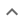•# Finding the roots of polynomials

We think you wrote:

###This solution deals with finding the roots (zeroes) of polynomials.

Solution found(y+2)*(y+1)*(y+3)

## Step by Step Solution## Step  1  :

#### Equation at the end of step  1  :

  (((y3)+5y)+(6•(y2)))+6•(y+1)
Step  2  :

#### Equation at the end of step  2  :

  (((y3) +  5y) +  (2•3y2)) +  6 • (y + 1)


## Step  3  :

#### Checking for a perfect cube :

y3+6y2+11y+6  is not a perfect cube

#### Trying to factor by pulling out :

Factoring:  y3+6y2+11y+6

Thoughtfully split the expression at hand into groups, each group having two terms :

Group 1:  11y+6
Group 2:  6y2+y3

Pull out from each group separately :

Group 1:   (11y+6) • (1)
Group 2:   (y+6) • (y2)

Bad news !! Factoring by pulling out fails :

The groups have no common factor and can not be added up to form a multiplication.

### Polynomial Roots Calculator :

Find roots (zeroes) of :       F(y) = y3+6y2+11y+6
Polynomial Roots Calculator is a set of methods aimed at finding values of  y  for which   F(y)=0

Rational Roots Test is one of the above mentioned tools. It would only find Rational Roots that is numbers  y  which can be expressed as the quotient of two integers

The Rational Root Theorem states that if a polynomial zeroes for a rational number  P/Q   then  P  is a factor of the Trailing Constant and  Q  is a factor of the Leading Coefficient

In this case, the Leading Coefficient is  1  and the Trailing Constant is  6.

The factor(s) are:

of the Leading Coefficient :  1

of the Trailing Constant :  1 ,2 ,3 ,6

Let us test ....

P  Q  P/Q  F(P/Q)   Divisor
-1     1      -1.00      0.00    y+1
-2     1      -2.00      0.00    y+2
-3     1      -3.00      0.00    y+3
-6     1      -6.00      -60.00
1     1      1.00      24.00
2     1      2.00      60.00
3     1      3.00      120.00
6     1      6.00      504.00

The Factor Theorem states that if P/Q is root of a polynomial then this polynomial can be divided by q*x-p Note that q and p originate from P/Q reduced to its lowest terms

In our case this means that
y3+6y2+11y+6
can be divided by 3 different polynomials,including by  y+3

### Polynomial Long Division :

Polynomial Long Division
Dividing :  y3+6y2+11y+6
("Dividend")
By         :    y+3    ("Divisor")

 dividend y3 + 6y2 + 11y + 6 - divisor * y2 y3 + 3y2 remainder 3y2 + 11y + 6 - divisor * 3y1 3y2 + 9y remainder 2y + 6 - divisor * 2y0 2y + 6 remainder 0

Quotient :  y2+3y+2  Remainder:  0

#### Trying to factor by splitting the middle term

Factoring  y2+3y+2

The first term is,  y2  its coefficient is  1 .
The middle term is,  +3y  its coefficient is  3 .
The last term, "the constant", is  +2

Step-1 : Multiply the coefficient of the first term by the constant   1 • 2 = 2

Step-2 : Find two factors of  2  whose sum equals the coefficient of the middle term, which is   3 .

 -2 + -1 = -3 -1 + -2 = -3 1 + 2 = 3 That's it

Step-3 : Rewrite the polynomial splitting the middle term using the two factors found in step 2 above,  1  and  2
y2 + 1y + 2y + 2

Step-4 : Add up the first 2 terms, pulling out like factors :
y • (y+1)
Add up the last 2 terms, pulling out common factors :
2 • (y+1)
Step-5 : Add up the four terms of step 4 :
(y+2)  •  (y+1)
Which is the desired factorization

## Final result :

  (y + 2) • (y + 1) • (y + 3)